American Journal of Modern Physics
Volume 5, Issue 4, July 2016, Pages: 45-50

Mass, Momentum, Force and Energy of Photon and Subatomic Particles, and Mechanism of Constant Light Speed Based on Yangton & Yington Theory

Edward T. H. Wu

Solar Buster Corporation, Los Angeles, USA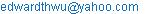Edward T. H. Wu. Mass, Momentum, Force and Energy of Photon and Subatomic Particles, and Mechanism of Constant Light Speed Based on Yangton & Yington Theory. American Journal of Modern Physics. Vol. 5, No. 4, 2016, pp. 45-50. doi: 10.11648/j.ajmp.20160504.11

Received: April 18, 2016; Accepted: April 27, 2016; Published: June 7, 2016

Abstract: The meanings of Mass, Momentum, Force and Energy of a substance are clearly defined. Their relationships to each others are discussed. The reasons that a Photon has energy E = hν and momentum P = h/λ are explained. Light travels in vacuum at a constant speed C = 3 x 108 m/sec while converting from Wu’s Particle through the separation and ejection processes from its parent substance is discussed in details based on Yangton and Yington theory. In Black Body radiation, a Photon emitted at high temperature having smaller size, higher frequency and higher energy is also explained.

Keywords: Mass, Force, Momentum, Energy, Yangton, Yington, Photon, Wu’s Particle, Higgs Boson, Electron, String Structure, Light Speed, Black Body Radiation

Contents

1. Introduction

Wikipedia defines Mass as follows: "In physics, Mass is a property of a physical body. It is generally a measure of an object's resistance to changing its state of motion when a force is applied. It is determined by the strength of its mutual gravitational attraction to other bodies, its resistance to acceleration or directional changes, and in the theory of relativity gives the mass–energy content of a system. The SI unit of mass is the kilogram (kg).

Mass is not the same as weight, even though we often calculate an object's mass by measuring its weight with a spring scale instead of comparing it to known masses. An object on the Moon would weigh less than it would on Earth because of the lower gravity, but it would still have the same mass. This is because weight is a force, while mass is the property that (along with gravity) causes this force.

In Newtonian physics, mass can be generalized as the amount of matter in an object. However, at very high speeds or for subatomic particles, special relativity shows that energy is an additional source of mass. Thus, any stationary body having mass has an equivalent amount of energy, and all forms of energy resist acceleration by a force and have gravitational attraction. In addition, "matter" is a loosely defined term in science, and thus cannot be precisely measured." .

We can summarize the above statements as follows:

Mass is proportional to Force and inversely proportional to acceleration .

Mass can be determined by Gravitational Force  which is proportional to the Masses of both objects and inversely proportional to the square of distance.

Mass is not Weight which is a Gravitational Force.

Mass is the amount of Matter in an object.

Energy is an additional source of Mass according to special relativity .

Since special relativity is ambiguous , we will only focus on the discussions on the definitions of Mass associated with Force, Gravitational Force and the amount of Matter.

Subatomic Particles  such as Wu’s Particle (a still Yangton and Yington Circulating Pair) , Higgs Boson , Electron, Neutron and Proton, all have String Structures  as proposed by Edward T. H. Wu , they follow exactly the same physical laws and principles like any other object. Photon (a free Yangton and Yington Circulating Pair) (Fig. 1) however is an exception. Why the Mass, Force, Momentum and Energy of a Photon  are different from that of other subatomic particles? Why the light speed in vacuum is always a constant? We will discuss these issues in details in this paper.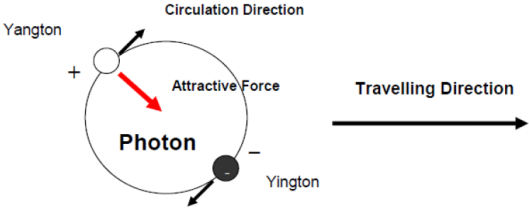Fig. 1. A Hypothetical Photon.

2. Mass

2.1. Definition of Mass

Mass (M) is the total amount of substance contained in an object. Because Wu’s Particle, a still Yangton and Yingtone circulating pair, is the building block of all substances, Mass (M) is also the measurement of the total amount of Wu’s Particles contained in an object.

2.2. Newton’s Second Law of Motion

Newton’s Second Law of Motion  states that the motion of an object can be influenced by Force based on the following formula:

F = MNewton’s a  (1)

In which, Newton’s Mass (MNewton’s) is a constant associated with an object rather than the total amount of the substance in the object. In another word, Newton’s Mass (MNewton’s) is defined as:

Mass (MNewton’s) = F/a

However, in Wu’s Yangton & Yington Theory, Mass (M) is the total amount of the substance which is also the total amount of Wu’s Particles (MWu’s) in the object.

3. Force

3.1. Definition of Force

Force is the intensity of influence applied on an object to cause change of motion.

3.2. Definition of Impulse

Impulse (dP) is the influence applied on an object by Force (F) in a finite time (dt)

dP= F x dt   (2)

3.3. Law of Force

The amount of impulse (dP) applied on an object by Force (F), in a finite time (dt) is F x dt. And the response of the object is the change of velocity (dV). Because of the linear relationship between impulse and response, therefore

dP = Mobj x dV   (3)

Also

F x dt = Mobj x dV   (4)

Where Mobj is a constant associated with the object.

Because,

F = (MobjdV)/dt = Mobj (dV/dt)

Therefore,

F = Mobja    (5)

Newton’s Second Law of Motion is nothing but a derivative of Law of Force. Both laws have constants associated with the object however there are no direct evidences that these constants are the amount of substance.

Only Wu’s Yangton and Yington Theory gives a clear definition and explanation that Mass is the total amount of substance (M) which is also the total amount of Wu’s Particles (MWu’s) contained in the object. With Wu’s Yangton and Yington Theory, Law of Force becomes an obvious consequence.

F x dt = M x dV    (6)

And

F = Ma   (7)

4. Momentum

Definition of Momentum

Momentum (P) is the total impulse applied on an object by Force (F) in a period of time (t):

P = ∑dP= ∑(F x dt)    (8)

Because of (2) & (6),

dP = F x dt = M x dV = d(MV)

∑dP = ∑d(MV)

Therefore,

P – P0 = MV – MV0

Also,

P0 = 0 & V0 = 0

Therefore,

P = MV    (9)

5. Energy

5.1. Law of Energy

Energy is the amount of the interaction applied on an object caused by the impulse and response. For mechanical Energy, the impulse on the object is the change of Momentum (dP) and the response is the change of speed (dV), therefore

dE= dP x dV    (10)

Because of (2),

dP = (F x dt) and dV = (dX/dt)

dE = (F x dt) (dX/dt) = F x dX

Therefore,

dE = FdX   (11)

5.2. Kinetic Energy

Because of (11) & (7),

dE = F dX = Ma dX = M (dV/dt) dX =MV dV

Therefore,

E – E0 = ½ M (V2 –V02)

Also,

E0 = 0, V0 = 0

Therefore,

Ek = ½ MV2   (12)

Because of (9),

P = MV

Therefore,

Ek = P2/2M   (13)

5.3. Potential Energy

In Gravitational Field, an object of Mass (M) has a Potential Energy associated with its position. The difference of the Potential Energy of an object between the final position and the initial position is equal to the work done by the external force moving the object from the initial position to the final position. Therefore,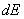=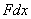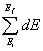=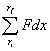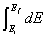=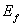-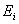Also,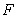=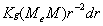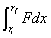=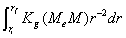=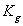(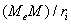-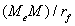)

Therefore,

Ef – Ei = KgMeM(1/ri – 1/rf)    (14)

Where Me is the Mass of Earth and Kg = 6.674×10−11 Nm2 kg-2.

5.4. Transformation of Energy

In Gravitational Field, an object of Mass (M) at distance (r) can gain a Kinetic Energy that is converted through the reduction of Potential Energy from infinitive (∞) to distance (r). Therefore,

½ MV2 = Kg (MeM)/r   (15)

6. Higgs Boson & Electron

According to Newton’s Law of Universal Gravitation , the Gravitational Force between two objects is

Fg = KgMg1Mg2/r2   (16)

Where Kg = 6.674×10−11 Nm2 kg-2

In which, the Gravitational Mass (Mg) is the amount of substances that responds to the Gravitational Force. Since all Subatomic Particles made of String Structures of Wu’s Particles such as Higgs Boson, Electron, Neutron, Proton, etc., can generate Gravitational Force, therefore Mg = MWu’s.

Because,

Ftotal = Fg

Also,

Ftotal = Mtotal a = MWu’s a = Mga

Therefore,

Fg = Mg  (17)

According to Coulomb’s Law , the Electrical Force between two charged objects is

Fe = KeQ1Q2/r2    (18)

Where Ke = 8.99×109 N m2 C−2

In which, the Electrical Charge (Q) is proportional to the amount of substances that responds to the Electrical Force (Melect). Because Melect is a portion of the total Mass (Mg), therefore

Melect < Mg

Therefore,

Ftotal = Fe = Mga > Melecta    (19)

7. Wu’s Particle

What is the Mass of a Wu’s Particle? Since Wu’s Particle contains only a Yangton and Yington circulating pair that is confined inside the substance, therefore the Mass of a Wu’s Particle is nothing but a pair of Yangton and Yington super fine particles.

Wu’s Particle is the basic building block of all matters. Its Mass, Momentum and Energy are a small portion of the entire substance that is made of String Structures such as Higgs Boson, Neutron, Proton and Electron, etc., and their interferences with Force are all governed by the same physical laws and principles.

8. Photon

What is the Mass of a Photon? Since Photon is a free Yangton and Yington circulating pair traveling in the vacuum, the Mass of a Photon is the same as that of Wu’s Particle. It is a pair of Yangton and Yington super fine particles.

Since Yangton and Yington are a pair of extremely fine particles with opposite Electric Unit-Charge such that any force applied on the Photon will be cancelled by the counter force, therefore Photon can’t be interfered by Gravitational Force or Electromagnetic Force .

Unlike Wu’s Particle which is a part of substance, the Momentum and Energy of a free Photon are generated in a two stage separation processes by releasing Wu’s Particle from its parent substance made of String Structure such as Higgs Boson, Electron, Neutron, Proton, etc. (Fig. 2). This two stage separation processes contains Separation Stage and Ejection Stage: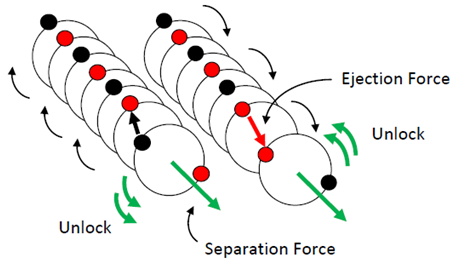Fig. 2. Photon Formed in a two stage processes by releasing Wu’s Particle from its Parent Substance.

A. Separation Stage

Like a whirlpool or a hurricane, the Separation Force needed to unlock a Wu’s Particle from the end of a String Structure is proportional to the frequency (ν) of the circulating Yangton and Yington pair (Fig. 2). Therefore,

Fseparation ∞ ν

Fseparation = k1ν

Where K1 is a constant.

Because of (2),

dP = Fdt = k1νdt

Therefore,

∆P = k1ν∆t

Since the separation time (∆t) is extremely small and it is the same for all Photons regardless of their frequencies,

Also,

∆P = P

Ks = K1∆t

Therefore,

P = ksν   (20)

Where Ks is a constant.

B.  Ejection Stage

The ejection force of a Photon from its parent substance is caused by the repulsive forces between the same particles, Yangton vs. Yangton and Yington vs. Yington, of the adjacent Wu’s Particles (Fig. 2). Therefore, the light speed of a Photon, regardless to its frequency, formed by the separation and ejection processes of Wu’s particle from its parent substance should always be a constant:

Light Speed (C) is a constant = 3 x 108 m/s    (21)

Because the Photon separation and ejection processes is a non-inertia process , once the Photon ejected away from the parent substance, it travels in vacuum at its own direction and constant light speed without any interference from its source.

Since Energy is the interaction between the interference and the response, therefore, in a small time interval, the Energy difference (∆E) is equal to the change of Momentum (∆P) multiple the change of Speed (∆V):

∆E = ∆P∆V

Because,

∆E = E, ∆P = P & ∆V = C

Therefore,

E = PC   (22)

Because of (20),

P = ksν

E = ksνC = (ksC)ν

Giving,

h = ksC

Then,

E = hν   (23)

Where h is Planck constant, h = 6.626 × 10-34 m2 kg / s

Because of (22) & (23),

P = E/C = (h/C)ν = h/(C/ ν)

Therefore,

P=h/λ     (24)

Where λ is the wavelength.

In summary, Table 1 shows the comparisons of Energy and Momentum between Photon and String Structures.

Table 1. Comparisons between Photon and String Structures.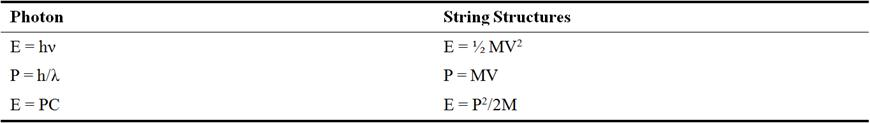9. Circulating Pair

Fig. 3 is a schematic picture of Wu’s Particle, a Yangton and Yington Circulating Pair. The central acceleration (ac) can be calculated as follows:

ac = dV/dt = (V1 dS/r)/dt = V1(dS/dt)/r = V2/r

Therefore,

Fc ∞ ac ∞ V2/r

Because,

F attraction ∞ 1/(2r)2

Also,

Fc = F attraction

Therefore,

V2/r = k/ 4r2

And,

V2r = k = constant   (25)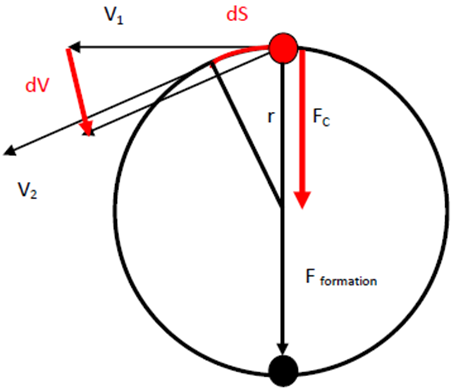Fig. 3. Theory of Circulating Pair.

Fig. 4 is the spectrum of Black Body Radiation  at different temperatures. It is obvious that at high temperature, the peak of the spectrum which corresponding to the amount of the majority Photons emitted from the Black Body has higher energy (hν) but smaller wave length (λ). This can be explained very well by the theory of Circulating Pair as follows:

When temperature (T) increases (↑), then circulation energy (E circulation) also increases (↑).

Because, E circulation = ½ MV2, velocity of circulation (V circulation) also increases (↑).

Because, V2r = constant, therefore, the radius of the circulation orbit (r) decreases (↓).

Because, circulating frequency (ν) = Vcirculation/2πr, therefore, frequency (ν) increases (↑).

Also, energy of Photon (E Photon = hν) increases (↑).

Because, νλ = C = constant, therefore, wave length (λ) decreases (↓).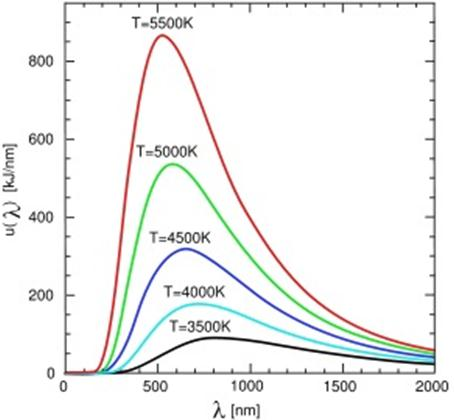11. Conclusion

Mass is the amount of substance, also the amount of Wu’s Particles in substance. Momentum is the total impulse caused by Force on an object. Force is the intensity of impulse with respect to time. Energy is the total interaction between impulse and response. And Law of Force states the linear relationship between the impulse and response of an object.

Wu’s Particle, a confined Yangton and Yington circulating pair, is the building block of all subatomic particles of String Structures such as Higgs Boson, Electron, Neutron and Proton, etc. It follows the basic physical laws and principles. Photon, on the other hand, is a free particle formed by the same Yangton and Yington circulating pair. The Momentum and Energy of a Photon are generated during the separation processes of Wu’s Particle from its parent substance. The separation processes contains two stages, Separation Process and Ejection Process. The separation force is proportional to the frequency of Yangton and Yington circulation which results in the Photon Energy E = hν. The ejection force on the other hand is generated by the repulsive forces between the same particles, Yangton vs. Yangton and Yington vs. Yington, of the adjacent Wu’s Particles. Therefore, the light speed, regardless to its frequency, should always be a constant. In Black Body radiation, a Photon emitted at high temperature has smaller size, smaller wave length, but higher frequency and higher energy which can be explained very well by Yangton and Yington Theory.

References

1. https://en.wikipedia.org/wiki/MassChandrasekhar, Subrahmanyan (2003). Newton's Principia for the common reader. Oxford: Oxford University Press. (pp. 1–2).
2. Andrew Motte translation of Newton's Principia (1687) Axioms or Laws of Motion.
3. Chandrasekhar, Subrahmanyan (2003). Newton's Principia for the common reader. Oxford: Oxford University Press. (pp. 1–2).
4. Einstein A. (1916), Relativity: The Special and General Theory (Translation 1920), New York: H. Holt and Company.
5. Edward T. H. Wu. Light Speed in Vacuum Is not a Constant and Time Doesn’t Change with Velocity – Discrepancies Between Relativities and Yangton & Yington Theory. American Journal of Modern Physics.Vol. 4, No. 6, 2015, pp. 367-373. doi: 10.11648/j.ajmp.20150406.12.
6. "Subatomic particles". NTD. Retrieved 5 June 2012.
7. Edward T. H. Wu, "Yangton and Yington – A Hypothetical Theory of Everything", Science Journal of Physics, Volume 2015, Article ID sjp-242, 6 Pages, 2015, doi: 10.7237/sjp/242.
8. http://en.wikipedia.org/wiki/Higgs_boson.
9. Polchinski, Joseph (1998). String Theory, Cambridge University Press ISBN 0521672295.
10. Edward T. H. Wu. Subatomic Particle Structures and Unified Field Theory Based on Yangton and Yington Hypothetical Theory. American Journal of Modern Physics. Vol. 4, No. 4, 2015, pp. 189-195. doi: 10.11648/j.ajmp.20150404.15.
11. Coulomb (1785a) "Premier mémoire sur l’électricité et le magnétisme,"Histoire de l’Académie Royale des Sciences, pages 569-577.
12. Loudon, R. (2000) . The Quantum Theory of Light (third ed.). Cambridge University Press. ISBN 0-19-850177-3.

 Contents 1. 2. 2.1. 2.2. 3. 3.1. 3.2. 3.3. 4. 5. 5.1. 5.2. 5.3. 5.4. 6. 7. 8. 9. 10. 11.
Article ToolsAbstractPDF(527K)